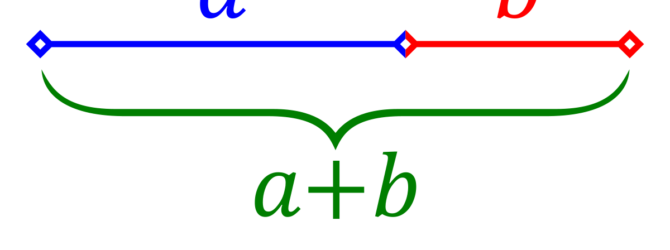# In mathematics, two quantities are in the golden ratio if…

The Math of God.
The article is bringing a couple of arguments about a Super Intelligent mind behind Science (Math and Logic especially) in the universe named God (Christian God).
The foundation of Science (Math, Logic…) always require a mind.
Mathematicians Cantor, Godel and Turin proved mathematically that human knowledge is limited.
God (defined as outside of time space and matter) is Logically. necessary.

If not, can you give us another logical and rational explanation?
If you can explain the origins of Logic you use, we are glad to see your arguments.
Thx

THE MATH OF GOD – phi

In mathematics, two quantities are in the golden ratio if their ratio is the same as the ratio of their sum to the larger of the two quantities. The figure on the right illustrates the geometric relationship. Expressed algebraically, for quantities a and b with a > b > 0,